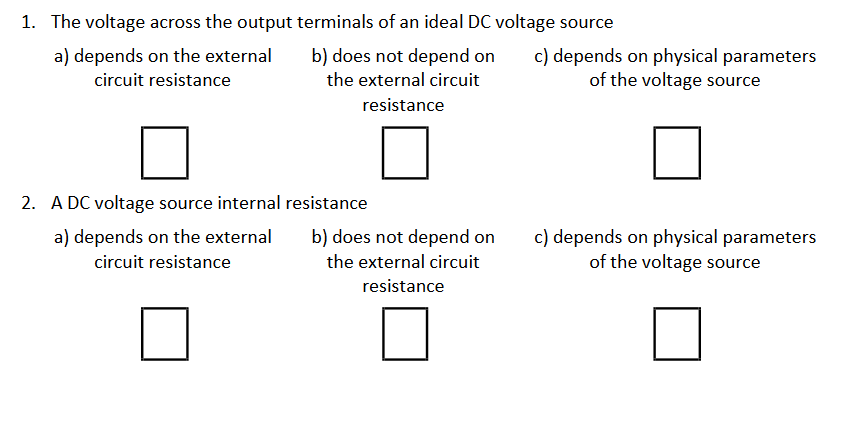# Question Solved1 Answer1. The voltage across the output terminals of an ideal DC voltage source a) depends on the external b) does not depend on c) depends on physical parameters circuit resistance the external circuit of the voltage source resistance 2. ADC voltage source internal resistance a) depends on the external b) does not depend on circuit resistance the external circuit resistance c) depends on physical parameters of the voltage sourceHCXQHC The Asker · Electrical EngineeringTranscribed Image Text: 1. The voltage across the output terminals of an ideal DC voltage source a) depends on the external b) does not depend on c) depends on physical parameters circuit resistance the external circuit of the voltage source resistance 2. ADC voltage source internal resistance a) depends on the external b) does not depend on circuit resistance the external circuit resistance c) depends on physical parameters of the voltage source
More
Transcribed Image Text: 1. The voltage across the output terminals of an ideal DC voltage source a) depends on the external b) does not depend on c) depends on physical parameters circuit resistance the external circuit of the voltage source resistance 2. ADC voltage source internal resistance a) depends on the external b) does not depend on circuit resistance the external circuit resistance c) depends on physical parameters of the voltage source